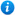* Watermarks do not appear on purchased resources

Preview resource: Click slides to enlarge

# Converting Units - Year 6

Converting Units - Year 6

Year 6 maths programme of study - Measurement:

• solve problems involving the calculation and conversion of units of measure, using decimal notation up to three decimal places where appropriate
• use, read, write and convert between standard units, converting measurements of length, mass, volume and time from a smaller unit of measure to a larger unit, and vice versa using decimal notation to up to three decimal places
• convert between miles and kilometres

'Converting Units - Year 6' is an ideal teaching aid to use during a lesson covering the year 6 curriculum objectives listed above. Content includes:

• Choosing the most appropriate metric unit of measure activity and worksheet
• Estimating measurements activity and worksheet
• Conversion factors recap
• Converting between units explanation
• Converting units of length activity and worksheet
• Converting units of volume activity and worksheet
• Converting units of mass activity and worksheet
• Converting units of time activity and worksheet
• Converting units reasoning and problem solving activity
• Calculating with metric units activity and worksheet
• Converting between miles and kilometres activity and worksheet

This animated maths PowerPoint presentation can be edited so teachers are able to adapt the resource to suit their individual teaching requirements.

Preview 'Converting Units - Year 6' in more detail by clicking on the PowerPoint images.

Our Price : £3.99 / 4 Credits